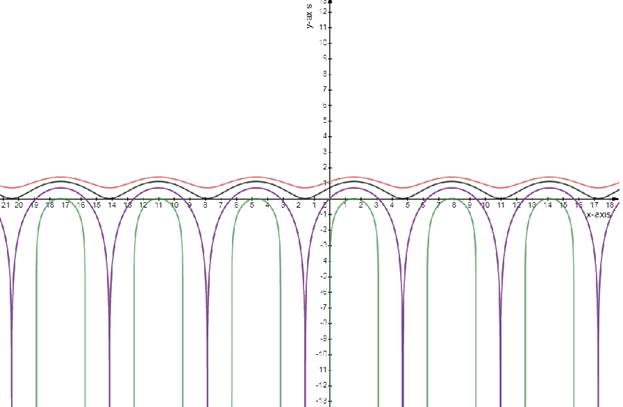# The features of the family members of the curve.### Single Variable Calculus: Concepts...

4th Edition
James Stewart
Publisher: Cengage Learning
ISBN: 9781337687805### Single Variable Calculus: Concepts...

4th Edition
James Stewart
Publisher: Cengage Learning
ISBN: 9781337687805

#### Solutions

Chapter 4, Problem 23RE
To determine

## The features of the family members of the curve.

Expert Solution

### Explanation of Solution

Given:

The function is

f(x)=ln(sinx+C)

Concept used:

The function is periodic with period of 2π with maximum at 2nπ+π2 .

Calculation:

The function is

f(x)=ln(sinx+C)................................(1)

The graph function will undefined

If C1 because (sinx+C) will become zero or negative and the natural logarithmic of zero or a negative number is undefined.

Using the value C=0,1,2and3

y=ln(sinx+0)y=ln(sinx+1)y=ln(sinx+2)y=ln(sinx+3)

The graph that the function is periodic with period of 2π with maximum at 2nπ+π2

For the function to be continuous on (,) the function has to be defined for any value of x

The function will have no graph when C1

When C the function becomes a straight line parallel to xaxis

Draw the graph

f(x)=ln(sinx+C)### Have a homework question?

Subscribe to bartleby learn! Ask subject matter experts 30 homework questions each month. Plus, you’ll have access to millions of step-by-step textbook answers!